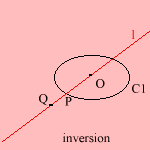# inverse

## main

When a curve C1 is given by a Cartesian function y = f(x), the inverse function is defined as the function g for which g(f(x)) = x. The corresponding inverse curve C2 has the same form as C1, only the x- and y-axis are interchanged.A more interesting case is polar inversion, where each point is inverted along the line through the center of inversion.
Given a curve C1, draw a line l through O. Line l intersects C1 in a point P. Now construct points Q of C2 so that OP * OQ = 1.

When curve C1 has been defined as r = f(φ), then the polar inverse - with O as the center of inversion - is a curve C2, which has as polar equation: r = 1 / f(φ). When C2 is the inverse of C1, then C1 is the inverse of C2.
Some curves have several curves inversely related to them. Each inverse then has a different center of inversion.

The first mathematician who discussed the curves was Steiner (1824).

A curve which is invariant under inversion - given a certain point of inversion - is called an anallagmatic curve 1). Examples include:

Moutard introduced the notion, in 1860.

An anallagmatic curve can be identified with a cyclic.

Other interesting inverse relations are the following.

 curve 1 center of inversion (curve 1) center of inversion (curve 2) curve 2 Archimedean spiral (parameter a) pole pole Archimedean spiral (parameter -a) Cayley's sextic focus Tschirnhausen's cubic circle center center circle cissoid (MacTutor: cardioid) cusp vertex parabola cochleoid pole - quadratrix conic focus pole limaçon ellipse focus pole or node ordinary limaçon epi spiral pole pole rhodonea Fermat's spiral pole pole lituus folium simple top of the knot Tschirnhausen's cubic hyperbola focus pole or node limaçon (with a noose) rectangular - center center lemniscate rectangular - vertex node (right) strophoid asymptote angle: φ/3 vertex node trisectrix of Maclaurin line not on line on circle circle parabola focus cusp cardioid sinusoidal spiral (parameter a) pole pole sinusoidal spiral (parameter -a) trisectrix of Maclaurin focus - Tschirnhausen's cubic

notes

1) Without change, from allagma (Gr.) = change.